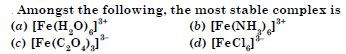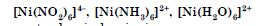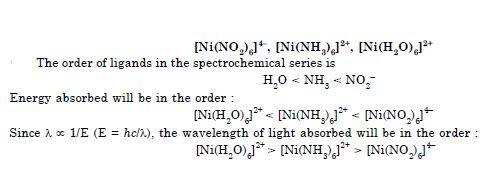# NCERT Solutions for Class 12 Chemistry Chapter 9 - Coordination Compounds

Get a clear understanding of the concept with the help of NCERT Solutions for Class 12. Designed in accordance with the latest syllabus, these solutions can help students achieve the marks they desire in the board exams. NCERT Solutions makes sure that important concepts are covered by the subject matter experts so that students can refer to them at any time they want.

The “Coordination Compounds” chapter of Class 12 Chemistry explains the unique properties of different compounds which are formed from transition metals, central atoms, isomerism in coordination compounds, polyhedron, oxidation numbers, bonding, ligands, coordination entity along with coordination number, etc.

##### Question 1:

On the basis of following observations made with aqueous solutions, assign secondary valencies in the following compounds.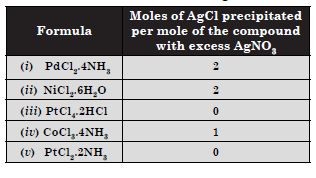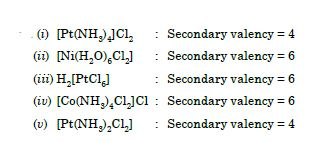##### Question 2:

Write the formulae of the following coordination compounds :

1. tetraammineaquachloridocobalt (III) chloride
2. potassium tetrahydroxozincate (II)
3. potassium trioxalatoaluminate (III)
4. dichloridobis (ethane-1,2-diamine) cobalt (III) ion
5. tetracarbonylnickel (0)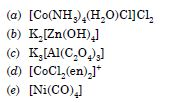##### Question 3:

Write the IUPAC names of the following coordination compounds :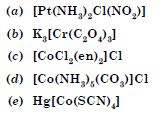1. diamminechloridonitrito-N-platinum (II)
2. potassium trioxalatochromate (III)
3. dichloridobis(ethane-1,2-diamine)cobalt (III) chloride
4. pentaamminecarbonatocobalt (III) chloride
5. Mercury tetrathiocyanatocobaltate (III)
##### Question 4:

Why is geometrical isomerism not possible in tetrahedral complexes having two different types of unidentate ligands coordinated with the central metal ion ?

Tetrahedral complexes do not show geometrical isomerism because the relative positions of the unidentate ligands attached to the central metal atom are the same with respect to each other.

##### Question 5:

Draw structures of geometrical isomers of [Fe(NH3)2(CN)4]–.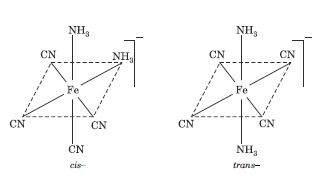##### Question 6:

Which out of the following two coordination entities is chiral (optically active)?

1. cis-[CrCl2(ox)2]3–
2. trans-[CrCl2(ox)2]3–

The two entities are represented as: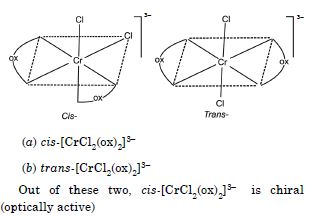##### Question 7:

The spin only magnetic moment of [MnBr4]2– is 5.9 B.M. Predict the geometry of the complex ion.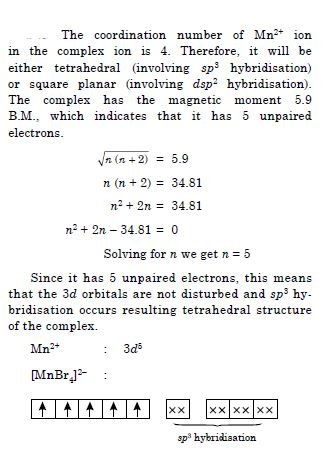##### Question 8:

Indicate the types of isomerism exhibited by the following complexes and draw the structures for these isomers :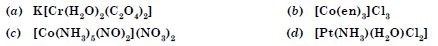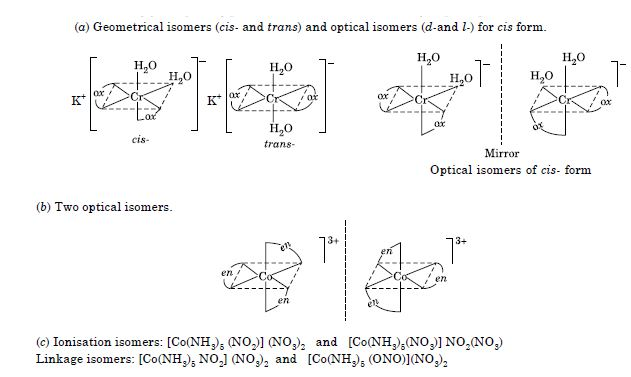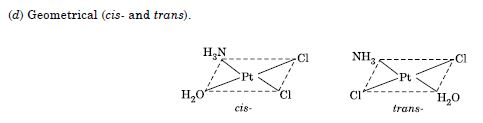##### Question 9: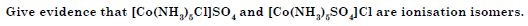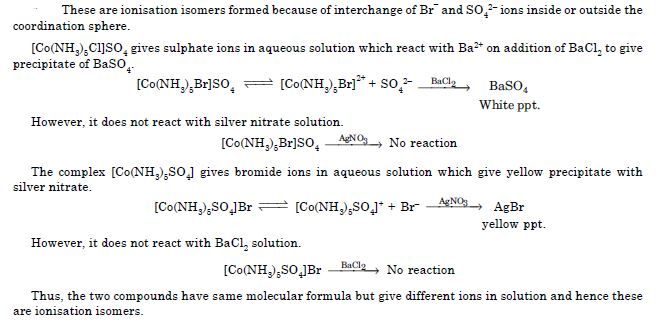##### Question 10:

Explain on the basis of valence bond theory that [Ni(CN)4]2– ion with square planar structure is diamagnetic and the [NiCl4]2– ion with tetrahedral geometry is paramagnetic.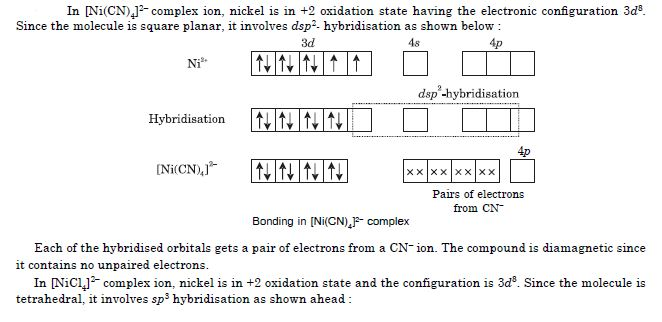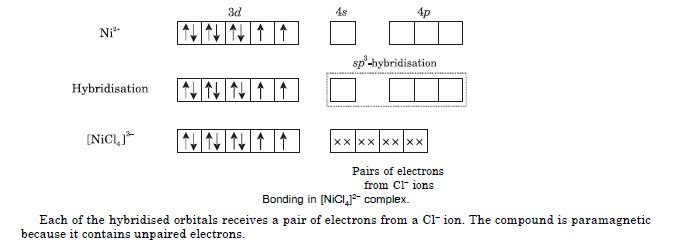##### Question 11: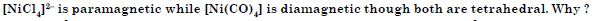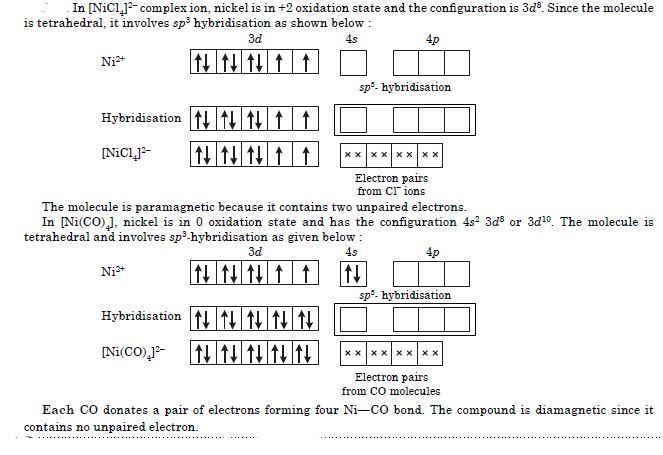##### Question 12: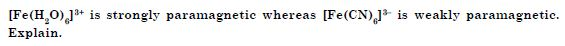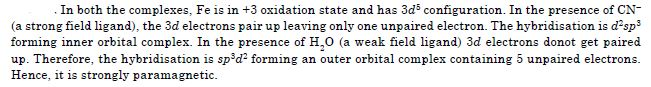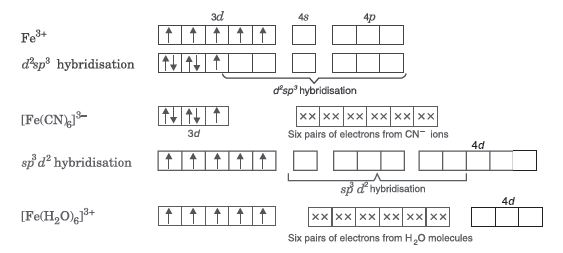##### Question 13: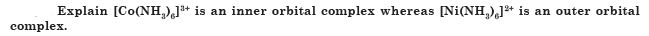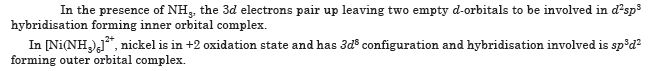##### Question 14:

The hexaaquomanganese(II) ion contains five unpaired electrons, while the hexacynoion contains only one unpaired electron. Explain using Crystal Field Theory.

Mn(II) has 3d5 electronic configuration. Water is a weak field ligand and therefore $∆$0 is small. Therefore, the hexaaqua complex will be high spin complex containing 5 unpaired electrons. On the other hand, CN– is a strong field ligand and therefore, $∆$0 is large. Therefore, in its cyano complex, the electrons pair up and have only one unpaired electron.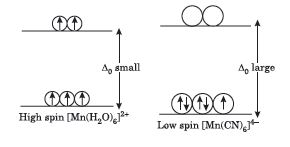##### Question 15:

Explain the bonding in coordination compounds in terms of Werner’s postulates.

The bonding in coordination compounds can be explained on the basis of Werner’s coordination theory. The main Postulates of Werner’s Coordination Theory are :

1. In coordination compounds, metal atoms exhibit two types of valencies namely, the primary valency and the secondary valency.
The primary valency is ionizable whereas the secondary valency is non-ionizable. In modern terminology, the primary valency corresponds to oxidation state and the secondary valency corresponds to coordination number.
2. Every metal atom has a fixed number of secondary valencies i.e., it has a fixed coordination number.
3. The metal atom tends to satisfy both its primary as well as secondary valencies. Primary valencies are satisfied by negative ions whereas secondary valencies are satisfied either by negative ions or by neutral molecules. In certain cases, a negative ion may satisfy both types of valencies.
4. The secondary valencies are always directed towards the fixed positions in space and this leads to definite geometry of the coordination compound. Based on primary (ionizable) and secondary (non-ionizable) valency, Werner represented the above complexes of cobalt (III) as: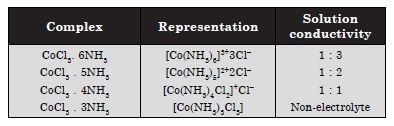##### Question 16: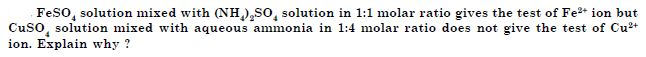##### Question 17:

Explain with two examples each of the following: coordination entity, ligand, coordination number, coordination polyhedron, homoleptic and heteroleptic.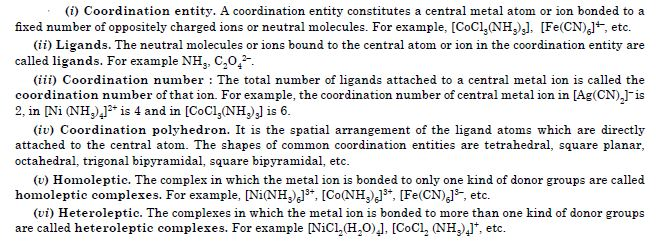##### Question 18:

What is meant by unidentate, didentate and ambidentate ligands? Give two examples for each.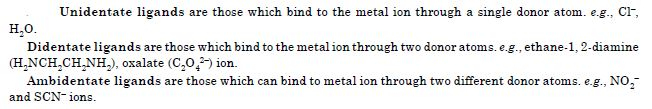##### Question 19: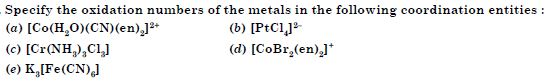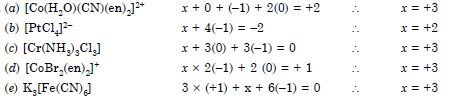##### Question 20:

Using IUPAC norms write the formulas for the following :

1. Tetrahydroxozincate (II)
2. Hexaamminecobalt (III) sulphate
4. Potassium tri (oxalato) chromate (III)
5. Diaminedichloridoplatinum (II)
6. Hexaammineplatinum (IV)
7. Potassium tetracyanonickelate (II)
8. Tetrabromidocuprate (II)
9. Pentaamminenitrito-O-cobalt (III)
10. Pentaamminenitrito-N-cobalt (III)##### Question 21: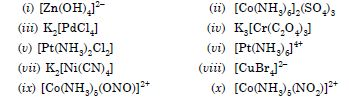1. Hexaamminecobalt (III) chloride
2. Tetraaminechloridonitrito-N-cobalt(III) chloride
3. Hexaamminenickel(II) chloride
4. Diamminechloridomethylamineplatinum(II) chloride
5. Hexaaquamanganese (II) ion
6. Tris (ethan-1,2-diamine)cobalt (III) ion
7. Hexaaquatitanium (III) ion
8. Tetrachloridonickelate (II) ion
9. Tetracarbonylnickel (0)
##### Question 22:

List various types of isomerism possible for coordination compounds, giving an example of each.

Two or more compounds having the same molecular formula but different arrangement of atoms are called isomers and the phenomenon is called isomerism. Isomers can be broadly classified into two major categories :

(A) Structural isomers (B) Stereoisomers.

(A) Structural isomers : The isomers which have same molecular formula but different structural arrangement of atoms or groups of atoms around the central metal ion are called structural isomers. These are of the following types :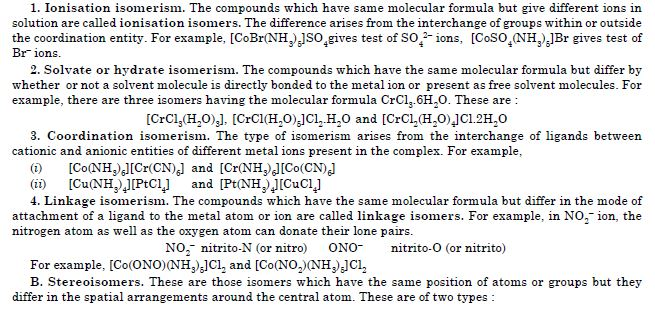1. Geometrical isomerism
Geometrical isomerism arises in complexes due to ligands occupying different positions around the central ion. The ligands occupy positions either adjacent to one another or opposite to one another. These are referred to as cis- form (ligands occupy adjacent positions) and trans- form (ligands occupy opposite positions).

(i) The geometrical isomerism is not possible in tetrahedral complexes. This is because in tetrahedral geometry all the positions are adjacent to one another in these complexes.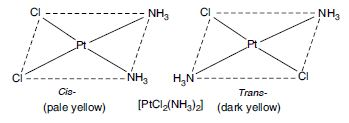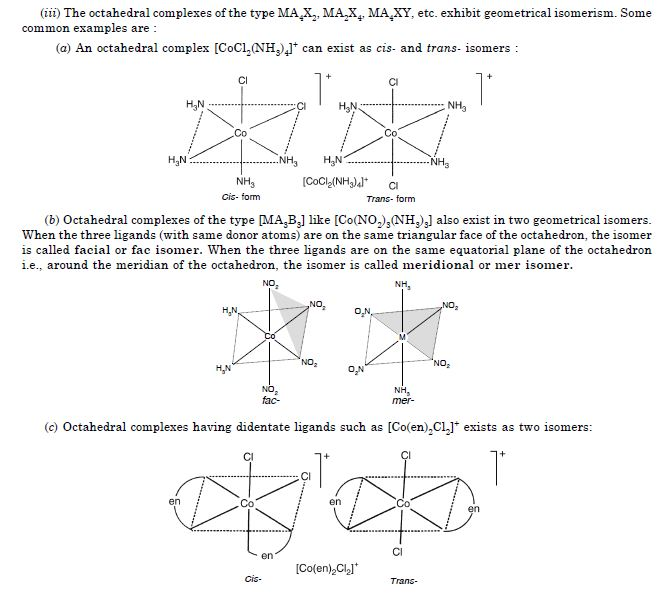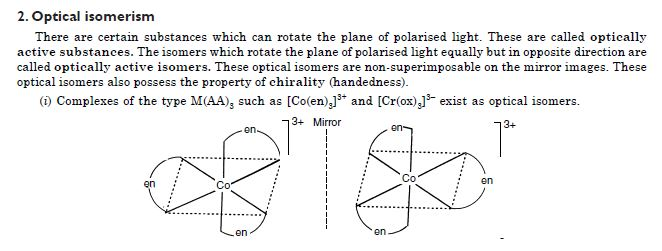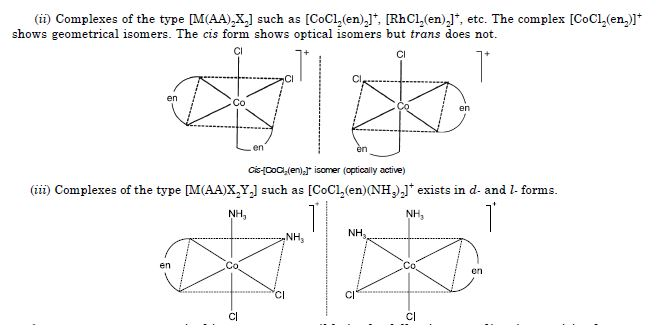##### Question 23: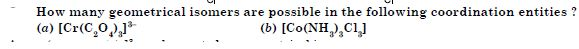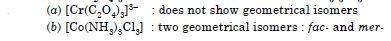##### Question 24: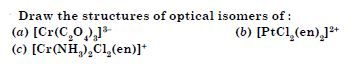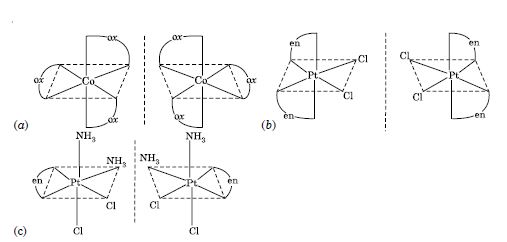##### Question 25: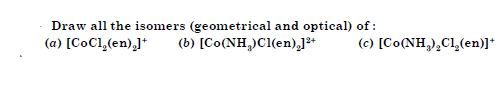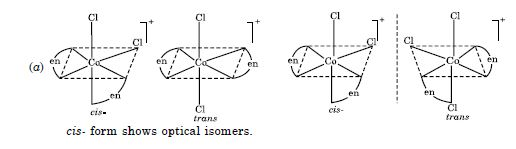##### Question 26:

Write all the geometrical isomers of [Pt(NH3)(Br)(Cl)(py)] and how many of these will exhibit optical isomers ?

Three isomers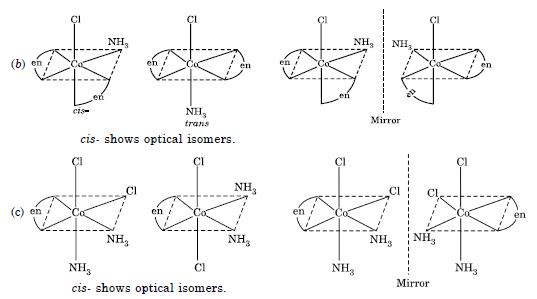These types of isomers donot show optical isomerism. Optical isomerism in square planar complexes occurs only with unsymmetrical chelating ligands.

##### Question 27:

Aqueous copper sulphate solution (blue in colour) gives :

1. a green precipitate with aqueous potassium fluoride and
2. a bright green solution with aqueous potassium chloride. Explain these experimental results.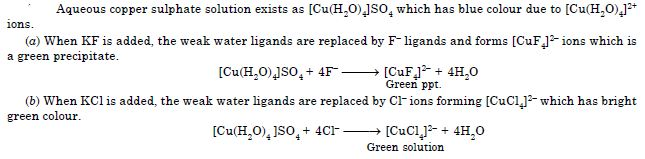##### Question 28:

What is the coordination entity formed when excess of aqueous KCN is added to an aqueous solution of copper sulphate ? Why is it that no precipitate of copper sulphide is obtained when H2S(g) is passed through this solution ?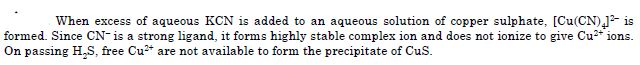##### Question 29:

Discuss the nature of bonding in the following coordination entities on the basis of valence bond theory: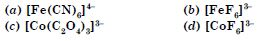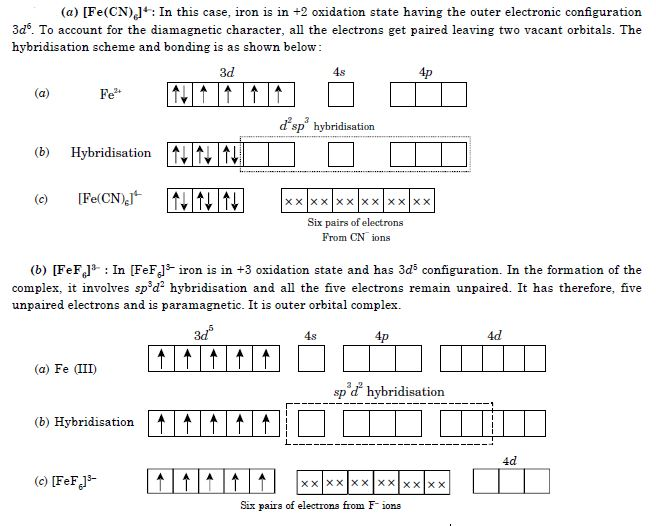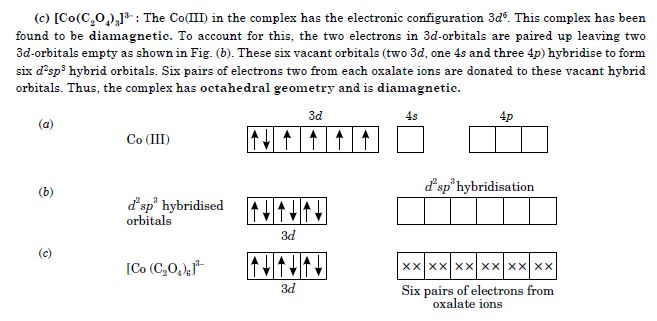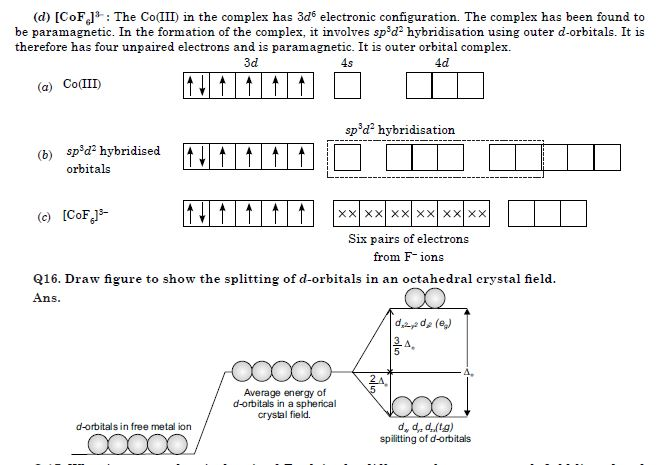##### Question 30:

Draw figure to show the splitting of d-orbitals in an octahedral crystal field.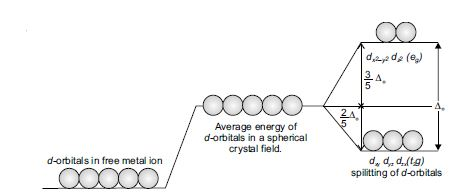##### Question 31:

What is spectrochemical series ? Explain the difference between a weak field ligand and a strong field ligand.

The arrangement of ligands in the increasing order of crystal field splitting is called spectrochemical series. This is shown below :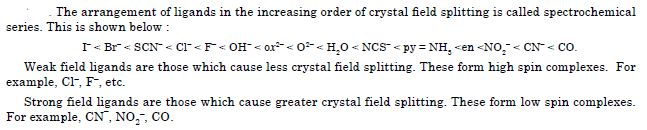##### Question 32:

What is crystal field splitting energy? How does the magnitude of $∆$0 decide the actual configuration of d-orbitals in a coordination entity ?

The conversion of five degenerate d-orbitals of the metal ion into different sets of orbitals having different energies in the presence of electrical field of ligands is called crystal field splitting. The crystal field splitting of d-orbitals in octahedral field is shown in Q.16.

The magnitude of $∆$0 helps to predict the actual configuration of the complex. If $∆$0 is large (as it is for strong field ligands), the electrons will try to remain in the lower set of orbitals. In this case, the complex has less number of unpaired electrons and is called low spin complex.

In this case, the repulsion due to pairing of electrons will be less than $∆$0.

On the other hand, if $∆$0 is small (as it is for weak field ligands), then after filling the lower set of three orbitals with one electron each, the next electron goes to the higher set of orbitals. In this case, the repulsion due to pairing of electrons will be more than $∆$0. The pairing of electrons will occur only after all the five orbitals are singly filled. In this case, the complex will have maximum number of unpaired electrons and is calld high spin complex.

Thus, the actual configuration adopted by the complex is decided by the relative values of $∆$0 and P where P represents the energy required for electron pairing in a single orbital.

For example, for d configuration.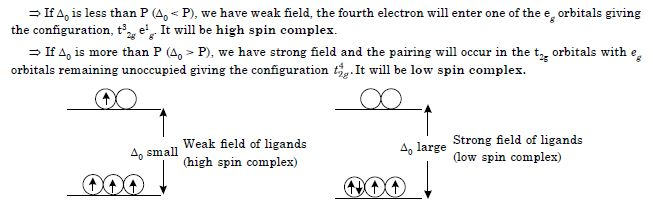##### Question 33: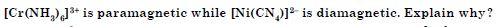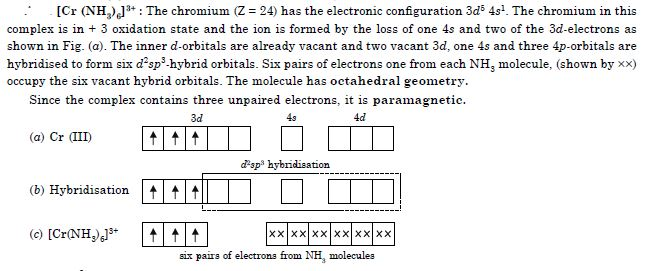##### Question 34: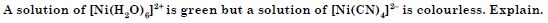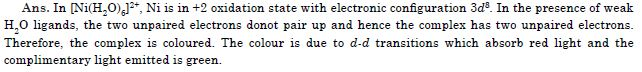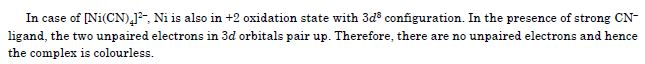##### Question 35:

[Fe(CN)6]4– and [Fe(H–2O)–6–]–2+– are of different colours in dilute solutions. Why?

In both these complexes iron is in +2 oxidation state having 3d6 electronic configuration. It has four unpaired electrons. In the presence of weak H2O ligand, these electrons donot pair up. However, in case of strong CN ligand, the electrons pair up leaving no unpaired electrons. Because of difference in number of unpaired electrons, these complexes have different colours.

##### Question 36:

Discuss the nature of bonding in metal carbonyls.

The bonding in metal carbonyls is described by the following steps :

1. There is a donation of lone pair of electrons of carbon (of CO) into the suitable empty orbital of the metal atom. This is a dative overlap and forms a sigma M $←$ C bond.
2. There is a $\mathrm{\pi }$-overlap involving donation of electrons from filled metal d-orbitals into vacant anti-bonding ${\mathrm{\pi }}^{*}$ molecular orbitals of CO. This results into the formation of M $\to$ C $\mathrm{\pi }$ bond. This is also called back donation or back bonding. The metal to ligand bonding creates a synergic effect which strengthens the bond between CO and the metal.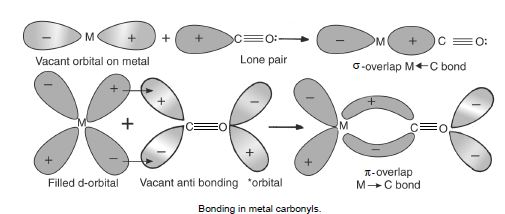##### Question 37:

Give the oxidation state, d-orbital occupation and coordination number of the central metal ion in the following complexes: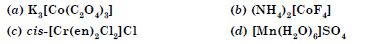##### Question 38:

Write down the IUPAC name for each of the following complexes and indicate the oxidation state, electronic configuration and coordination number. Also give stereochemistry and magnetic moment of the complex :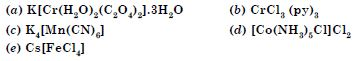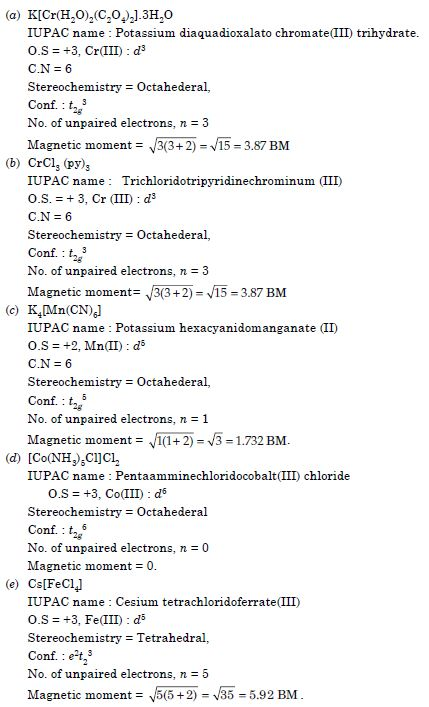##### Question 39: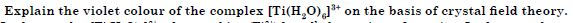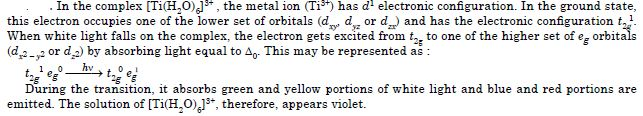##### Question 40:

What is meant by the chelate effect ? Give an example.

Chelate effect : The stability of a complex depends upon the formation of chelate rings. If the ligand, L is an unidentate ligand and L–L, a didentate ligand and if the donor atoms of L and L–L are the same element, then L–L will replace L. This stabilisation due to the chelation is called chelate effect. The enhanced stability of complexes containing chelating ligands is of great importance in biological systems and analytical chemistry. The chelate effect is maximum for 5- and 6-membered rings. In general, rings provide greater stability to the complex.

##### Question 41:

Discuss briefly giving an example in each case the role of coordination compounds in :

(a) biological system, (b) analytical chemistry, (c) medicinal chemistry and (d) extraction/metallurgy of metals.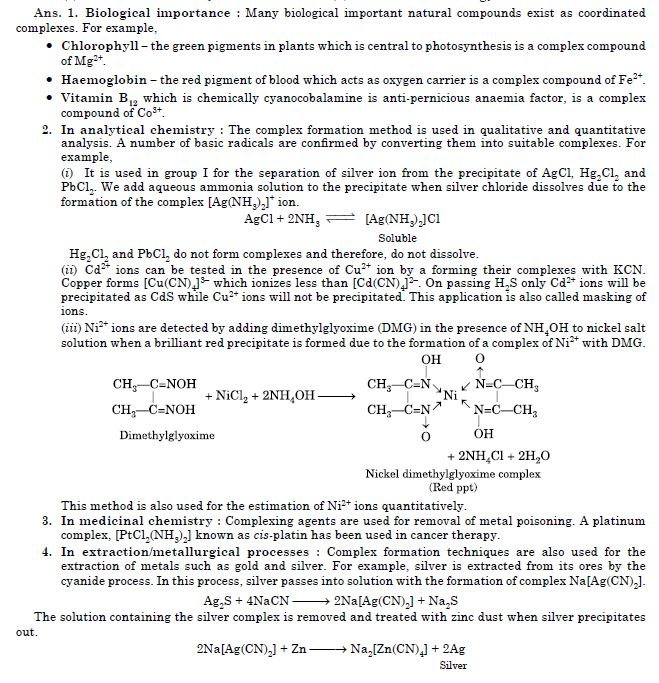Similarly, the cyanide process is used for the extraction of gold.
Similarly, purification of metals can also be achieved through formation nd subsequent decomposition of their coordination compounds. For example, impure nickel is converted to [Ni(CO)4], which is decomposed to get pure nickel.

##### Question 42: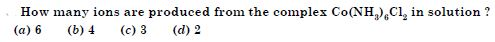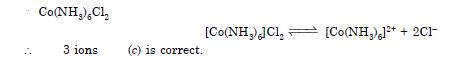##### Question 43: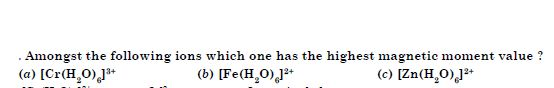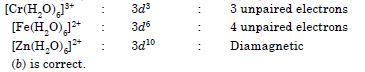##### Question 44:

The oxidation number of cobalt in K[Co(CO)4] is

1. +1
2. +3
3. –1
4. –3

K[Co(CO)4]
+1 + x + 4(0) = 0 $\therefore$ x = –1 (c) is correct.

##### Question 45: